# Bilateral Diagrams

The meaning of the diagrams below has been explained elsewhere. Here I only collected the simplest configurations for the reference sake.

 Some x exist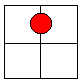No x existSome x' exist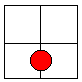No x' existSome y existNo y existSome y' exist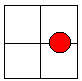No y' existSome xy exist    = Some x are y    = Some y are xNo xy exist    = No x are y    = No y are xSome x'y exist    = Some x' are y    = Some y are x'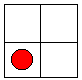No x'y exist    = No x' are y    = No y are x'Some x'y' exist    = Some x' are y'    = Some y' are x'No x'y' exist    = No x' are y'    = No y' are x'Some xy' exist    = Some x are y'    = Some y' are xNo xy' exist    = No x are y'    = No y' are xAll x are yAll x are y'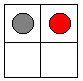All x' are y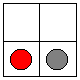All x' are y'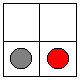All y are x existAll y are x'All y' are xAll y' are x'Some x are y,some are y'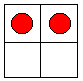Some x' are y,some are y'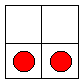Some y are x,some are x'Some y' are x,some are x'### Lewis Carroll